# JavaScript Function Generator

## JavaScript Function Generator

In this article, I am going to discuss JavaScript Function Generator with Examples. Please read our previous article where we discussed JavaScript Output in detail. As part of this article, we are going to discuss the following important pointers.

1. When do we use generators
2. What is Generator Function
4. Generator Instance methods
5. Example Fibonacci generator with next() method
6. Example Fibonacci generator without next() method
7. Example with Passing arguments to Generators
8. Example with return statement in a generator
9. Example Generator as an object property
10. Example Generator as an Object Method
11. Example Generator as a Complex Property
12. Example Generator defined in expression (anonymous function)
13. Example Generator with endless loop

JavaScript function* Keyword

Since ECMAScript 2015, a shorter syntax for declaring method definitions on objects initializers is introduced. It is a short cut for a function assigned to the method’s name.

The function* is an inbuilt JavaScript keyword that is used to define a generator function inside an expression. Generators are combination of functions and iterators.

##### Generator Properties:
• Generators are created by the generator function function* f(){}.
• Generators do not execute its body immediately when they are called.
• Generators can stop its execution midway and continue their executions from same point where it paused. The yield statement pauses the execution of a generator and returns a value.
• Generators are iterable so you can use them with the for…of loop.

Generator functions can be defined using the shorthand syntax as well.

###### When doing so:

Firstly, the asterisk (*) in the shorthand syntax must be after the function keyword. That is, function* foo(){} , here asterisk denoted that the foo() is a generator and not a normal function.

Secondly the yield statements return a value and pause the execution of the function. Non-generator function cannot contain the yield keyword. Always use yield in conjunction with the asterisk (*).

Syntax:
function* [name]([param1[, param2[, …, paramN]]]) {
statements
}

Parameters: This function accepts the following parameter as stated above and elaborated below:

• name: This the function name.
• paramN: This is the name of an argument to be passed to the function.
• statements: These are the body of the function.
###### Example-1:
```<html>
<title>JavaScript function* generator example.</title>
<body>
<h3>function* generatorHTML example Web Page</h3>
<p>Paragraph Section</p>

<script>
function* foo() {
let index = 0;
while (true) {
yield index++;
}
}

var f = foo();
console.log(f.next()); // 0
console.log(f.next()); // 1
console.log(f.next()); // 2
</script>
</body>
</html>```

Output: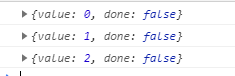In the above example we initialize the generator with var f = foo();  then we start the iterator on our generator f.next()); as soon as the first iteration starts the iterator. The code will return this object  {value:0, done: false}

The above output contains the value returning the output but why the done property is coming false for every value that will see in the 2nd example.

###### Example-2: Another generator function with yield along with return statement
```<html>
<title>JavaScript function* generator with return example. </title>
<body>
<h3>function* generator with return HTML example Web Page</h3>
<p>Paragraph Section</p>

<script>
function* generator(e) {
yield e + 10;
return 6;
yield e + 20;
yield e + 30;
}

var g = generator(24);
console.log(g.next());
console.log(g.next());
console.log(g.next());
</script>
</body>
</html>```

Output: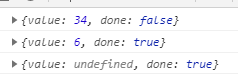A return statement in a generator will make the generator finish its execution like every other function. The done property of the generator object will be set to true and the value returned will be set to the value property of the generator object. All other yields will return undefined.

Memory Efficient: Generators are memory efficient as they required very less space for storing the value as they take values only according to their needs.

Generators don’t provide random access to element: Like arrays. As the values are iterated using yielded one by one based on call, we cannot access random elements.

Generators provide one-time access to element: Generators don’t allow us to iterate the function values again and again. Once all the values are traversed, we need to create a new Generator instance to iterate all the values again.

##### When do we use generators?

It is useful when working with asynchronous operation and when dealing with iterators or iterate through on demand-item loop means load when needed.

##### What is Generator Function?

Generator functions are the function that returns a multiple value at different interval and as per the user demands. The pause and resume are done using yield & next

Generator calculate their yielded values on demand. The next() method also accepts the value, which is used to modify the internal state of the generator. A value passed by next() method is received by yield.

The yield keyword stops the execution of the generator function and the value of the yield expression is returned to the generator’s caller.

The yield keyword returns an IteratorResult object with two properties, value and done.

When we hold on a yield expression, the generator’s code execution remains to stopped till the generator’s next() method is called. Each time the generator’s next() method is called, the generator restarts the execution, where the execution was paused and return the iterator result.

##### Generator Instance methods:
1. Generator.prototype.next(): It returns a value yielded by the yield expression.
2. Generator.prototype.return(): It returns the given value and finishes the generator.
3. Generator.prototype.throw(): It throws an error to a generator (also finishes the generator, unless caught from within that generator).
##### To understand more clearly let’s dig more into it.

So, when we called a generator function, it does not execute its body immediately instead of it returned an iterator object for the function. When the iterator’s next() method is called, the generator function’s body is executed until the first yield expression found, which denotes the value to be returned from the iterator or, with yield*, delegates/assign to another generator function.

The next() method returns an IteratorResult object with a value property containing the yielded value and a done property is a Boolean value which indicates whether the generator has yielded its last value.

When Passing an argument into Generator, on calling the next() method with an argument will resume the generator function execution, where an execution was paused by replacing the yield expression with the argument from next().

A return statement when executed, in a generator, it returns the given value and finishes the generator execution i.e. the done property of the IteratorResult object will be set to true and If any value is being returned, it will be set as the value property of the object returned by the generator. Same as a return statement, whenever an error is thrown inside the generator will make the generator finished — unless caught within the generator’s body.

We can force the generator to throw an exception by calling its throw() method and passing the exception value it should throw. If the exception is not caught from within the generator, it will pass on through the call to throw() and following calls to next() will return in the output done property with true

When a generator is finished, others next() method calls which are there in pipeline will not execute any of further generator’s code, they will simply return an object of in this form:

{value: undefined, done: true}.

##### Example Fibonacci generator with next() method:
```<html>
<title>JavaScript fibonaci Generator with next() method example.</title>
<body>
<script>
function* fibonacci() {
let fn1 = 0;
let fn2 = 1;
while (true) {
let current = fn1;
fn1 = fn2;
fn2 = current + fn1;
let reset = yield current;
if (reset) {
fn1 = 0;
fn2 = 1;
}
}
}
const sequence = fibonacci();
console.log(sequence.next().value);     // 0
console.log(sequence.next().value);     // 1
console.log(sequence.next().value);     // 1
console.log(sequence.next().value);     // 2
console.log(sequence.next().value);     // 3
console.log(sequence.next().value);     // 5
console.log(sequence.next().value);     // 8
console.log(sequence.next(true).value); // 0
console.log(sequence.next().value);     // 1
console.log(sequence.next().value);     // 1
console.log(sequence.next().value);     // 2
</script>
</body>
</html>```

Output: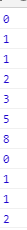##### Example Fibonacci generator without next() method:
```<html>
<title>JavaScript fibonaci Generator without next() method example</title>
<body>
<script>
function* fiboGen(len, current = 0, next = 1) {
if (len === 0) {
return current;
}
yield current;
yield* fiboGen(len - 1, next, current + next);
}
const fibo10 = [...fiboGen(10)];
console.log(fibo10)
//[0,1,1,2,3,5,8,13,21,34]

</script>
</body>
</html>```

Output: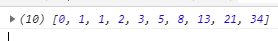##### Example simple Generator:
```<html>
<title>JavaScript simple generator expression example. </title>
<body>
<script>
function* simpleGen() {
var index = 0;
while (true)
yield index++;
}

var gen = simpleGen();

console.log(gen.next().value); // 0
console.log(gen.next().value); // 1
console.log(gen.next().value); // 2
console.log(gen.next().value); // 3
</script>
</body>
</html>```

Output:##### Example with yield* to delegate/assign to another generator function:
```<html>
<title>JavaScript yield* to delegate/assign to another generator function example. </title>
<body>
<script>
function* yieldstarGenerator(i) {
yield i + 1;
yield i + 2;
yield i + 3;
}

function* generator(i) {
yield i;
yield* yieldstarGenerator(i);
yield i + 10;
}

var gen = generator(10);

console.log(gen.next().value); // 10
console.log(gen.next().value); // 11
console.log(gen.next().value); // 12
console.log(gen.next().value); // 13
console.log(gen.next().value); // 20
</script>
</body>
</html>```

Output: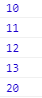##### Example with Passing arguments to Generators:
```<html>
<title>JavaScript Passing arguments to Generators example. </title>
<body>
<script>
function* argGenerator() {
console.log(0);
console.log(1, yield);
console.log(2, yield);
console.log(3, yield);
}

var gen = argGenerator();

// the first call of next executes from the start of the function
// until the first yield statement
gen.next();             // 0
gen.next('india');    // 1 india
gen.next('mumbai'); // 2 mumbai
gen.next('maharashtra'); // 3 maharashtra
</script>
</body>
</html>```

Output: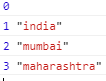##### Example with return statement in a generator:
```<html>
<title>JavaScript return statement in a generator example. </title>
<body>
<script>
function* yieldReturnGen() {
yield "Yield";
return "Return";
yield "not declared";
}

var gen = yieldReturnGen()
console.log(gen.next()); // { value: "Yield", done: false }
console.log(gen.next()); // { value: "Return", done: true }
console.log(gen.next()); // { value: undefined, done: true }
</script>
</body>
</html>```

Output: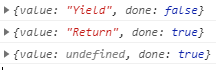##### Example Generator as an object property:
```<html>
<title>JavaScript Generator as an object property: example. </title>
<body>
<script>
const ObjGen = {
*generator() {
yield 'alpha';
yield 'beta';
}
}
const gen = ObjGen.generator()
console.log(gen.next()); // { value: 'alpha', done: false }
console.log(gen.next()); // { value: 'beta', done: false }
console.log(gen.next()); // { value: undefined, done: true }
</script>
</body>
</html>```

Output: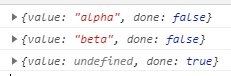##### Example Generator as an Object Method:
```<html>
<title>JavaScript Generator as an object method: example. </title>
<body>
<script>
class smaple {
*generator() {
yield 10;
yield 20;
yield 30;
}
}
const s = new sample();
const gen = s.generator();

console.log(gen.next()); // { value: 10, done: false }
console.log(gen.next()); // { value: 20, done: false }
console.log(gen.next()); // { value: 30, done: false }
console.log(gen.next()); // { value: undefined, done: true }
</script>
</body>
</html>```

Output: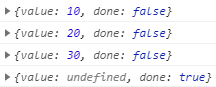##### Example Generator as a Complex Property:

about *[Symbol.iterator]() will discuss in upcoming topic

```<html>
<title>JavaScript Generator as a Complex property: example. </title>
<body>
<script>
class sample {
*[Symbol.iterator]() {
yield 10;
yield 20;
}
}

const SomeObj = {
*[Symbol.iterator]() {
yield 'alpha';
yield 'beta';
}
}

console.log(Array.from(new sample)); // [ 10, 20 ]
console.log(Array.from(SomeObj)); // [ 'alpha', 'beta' ]
</script>
</body>
</html>```

Output: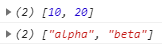##### Example Generator defined in expression (anonymous function):
```<html>
<title>JavaScript Generator defined in expression (anonymous function) example. </title>
<body>
<script>
const sample = function* () {
yield 10.1;
yield 20.3;
};

const sam = sample();
console.log(sam.next()); // {value: 10.1, done: false}
console.log(sam.next()); // {value: 20.3, done: false}
console.log(sam.next()); // {value: undefined, done: true}
</script>
</body>
</html>```

Output: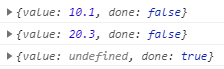##### Example Generator with endless loop:
```<html>
<title>JavaScript Generator with endless loop example. </title>
<body>
<script>
function* powers(n) {
//endless loop to generate
for (let current = n; ; current *= n) {
yield current;
}
}

for (let pow of powers(3)) {
//controlling generator
if (pow > 243) break;
console.log(pow)
//3
//9
//27
//81
//243
}
</script>
</body>
</html>```

Output: